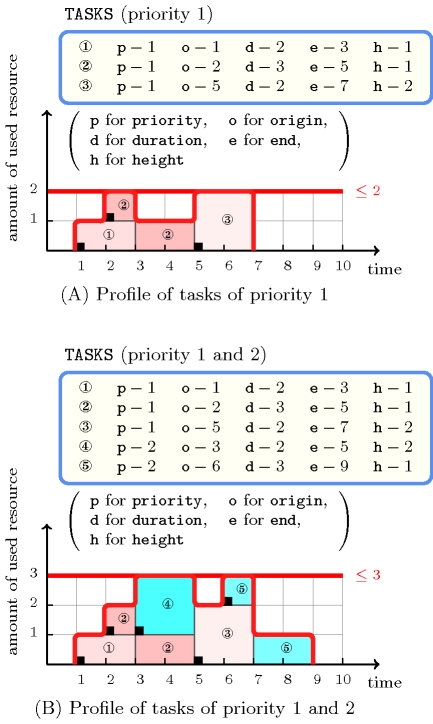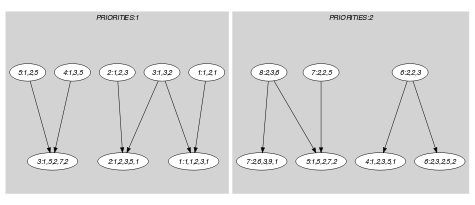## 5.100. cumulative_with_level_of_priority

Origin

H. Simonis

Constraint

$\mathrm{𝚌𝚞𝚖𝚞𝚕𝚊𝚝𝚒𝚟𝚎}_\mathrm{𝚠𝚒𝚝𝚑}_\mathrm{𝚕𝚎𝚟𝚎𝚕}_\mathrm{𝚘𝚏}_\mathrm{𝚙𝚛𝚒𝚘𝚛𝚒𝚝𝚢}\left(\mathrm{𝚃𝙰𝚂𝙺𝚂},\mathrm{𝙿𝚁𝙸𝙾𝚁𝙸𝚃𝙸𝙴𝚂}\right)$

Arguments
 $\mathrm{𝚃𝙰𝚂𝙺𝚂}$ $\mathrm{𝚌𝚘𝚕𝚕𝚎𝚌𝚝𝚒𝚘𝚗}\left(\begin{array}{c}\mathrm{𝚙𝚛𝚒𝚘𝚛𝚒𝚝𝚢}-\mathrm{𝚒𝚗𝚝},\hfill \\ \mathrm{𝚘𝚛𝚒𝚐𝚒𝚗}-\mathrm{𝚍𝚟𝚊𝚛},\hfill \\ \mathrm{𝚍𝚞𝚛𝚊𝚝𝚒𝚘𝚗}-\mathrm{𝚍𝚟𝚊𝚛},\hfill \\ \mathrm{𝚎𝚗𝚍}-\mathrm{𝚍𝚟𝚊𝚛},\hfill \\ \mathrm{𝚑𝚎𝚒𝚐𝚑𝚝}-\mathrm{𝚍𝚟𝚊𝚛}\hfill \end{array}\right)$ $\mathrm{𝙿𝚁𝙸𝙾𝚁𝙸𝚃𝙸𝙴𝚂}$ $\mathrm{𝚌𝚘𝚕𝚕𝚎𝚌𝚝𝚒𝚘𝚗}\left(\mathrm{𝚒𝚍}-\mathrm{𝚒𝚗𝚝},\mathrm{𝚌𝚊𝚙𝚊𝚌𝚒𝚝𝚢}-\mathrm{𝚒𝚗𝚝}\right)$
Restrictions
 $\mathrm{𝚛𝚎𝚚𝚞𝚒𝚛𝚎𝚍}$$\left(\mathrm{𝚃𝙰𝚂𝙺𝚂},\left[\mathrm{𝚙𝚛𝚒𝚘𝚛𝚒𝚝𝚢},\mathrm{𝚑𝚎𝚒𝚐𝚑𝚝}\right]\right)$ $\mathrm{𝚛𝚎𝚚𝚞𝚒𝚛𝚎}_\mathrm{𝚊𝚝}_\mathrm{𝚕𝚎𝚊𝚜𝚝}$$\left(2,\mathrm{𝚃𝙰𝚂𝙺𝚂},\left[\mathrm{𝚘𝚛𝚒𝚐𝚒𝚗},\mathrm{𝚍𝚞𝚛𝚊𝚝𝚒𝚘𝚗},\mathrm{𝚎𝚗𝚍}\right]\right)$ $\mathrm{𝚃𝙰𝚂𝙺𝚂}.\mathrm{𝚙𝚛𝚒𝚘𝚛𝚒𝚝𝚢}\ge 1$ $\mathrm{𝚃𝙰𝚂𝙺𝚂}.\mathrm{𝚙𝚛𝚒𝚘𝚛𝚒𝚝𝚢}\le |\mathrm{𝙿𝚁𝙸𝙾𝚁𝙸𝚃𝙸𝙴𝚂}|$ $\mathrm{𝚃𝙰𝚂𝙺𝚂}.\mathrm{𝚍𝚞𝚛𝚊𝚝𝚒𝚘𝚗}\ge 0$ $\mathrm{𝚃𝙰𝚂𝙺𝚂}.\mathrm{𝚘𝚛𝚒𝚐𝚒𝚗}\le \mathrm{𝚃𝙰𝚂𝙺𝚂}.\mathrm{𝚎𝚗𝚍}$ $\mathrm{𝚃𝙰𝚂𝙺𝚂}.\mathrm{𝚑𝚎𝚒𝚐𝚑𝚝}\ge 0$ $\mathrm{𝚛𝚎𝚚𝚞𝚒𝚛𝚎𝚍}$$\left(\mathrm{𝙿𝚁𝙸𝙾𝚁𝙸𝚃𝙸𝙴𝚂},\left[\mathrm{𝚒𝚍},\mathrm{𝚌𝚊𝚙𝚊𝚌𝚒𝚝𝚢}\right]\right)$ $\mathrm{𝙿𝚁𝙸𝙾𝚁𝙸𝚃𝙸𝙴𝚂}.\mathrm{𝚒𝚍}\ge 1$ $\mathrm{𝙿𝚁𝙸𝙾𝚁𝙸𝚃𝙸𝙴𝚂}.\mathrm{𝚒𝚍}\le |\mathrm{𝙿𝚁𝙸𝙾𝚁𝙸𝚃𝙸𝙴𝚂}|$ $\mathrm{𝚒𝚗𝚌𝚛𝚎𝚊𝚜𝚒𝚗𝚐}_\mathrm{𝚜𝚎𝚚}$$\left(\mathrm{𝙿𝚁𝙸𝙾𝚁𝙸𝚃𝙸𝙴𝚂},\mathrm{𝚒𝚍}\right)$ $\mathrm{𝚒𝚗𝚌𝚛𝚎𝚊𝚜𝚒𝚗𝚐}_\mathrm{𝚜𝚎𝚚}$$\left(\mathrm{𝙿𝚁𝙸𝙾𝚁𝙸𝚃𝙸𝙴𝚂},\mathrm{𝚌𝚊𝚙𝚊𝚌𝚒𝚝𝚢}\right)$
Purpose

Consider a set $𝒯$ of tasks described by the $\mathrm{𝚃𝙰𝚂𝙺𝚂}$ collection where each task has a given priority chosen in the range $\left[1,\mathrm{𝙿𝚁𝙸𝙾𝚁𝙸𝚃𝙸𝙴𝚂}\right]$. Let ${𝒯}_{i}$ denote the subset of tasks of $𝒯$ that all have a priority less than or equal to $i$. For each set ${𝒯}_{i}$, the $\mathrm{𝚌𝚞𝚖𝚞𝚕𝚊𝚝𝚒𝚟𝚎}_\mathrm{𝚠𝚒𝚝𝚑}_\mathrm{𝚕𝚎𝚟𝚎𝚕}_\mathrm{𝚘𝚏}_\mathrm{𝚙𝚛𝚒𝚘𝚛𝚒𝚝𝚢}$ constraint forces that at each point in time, the cumulated height of the set of tasks that overlap that point, does not exceed a given limit. A task overlaps a point $i$ if and only if (1) its origin is less than or equal to $i$, and (2) its end is strictly greater than $i$. Finally, it also imposes for each task of $𝒯$ the constraint $\mathrm{𝚘𝚛𝚒𝚐𝚒𝚗}+\mathrm{𝚍𝚞𝚛𝚊𝚝𝚒𝚘𝚗}=\mathrm{𝚎𝚗𝚍}$.

Example
$\left(\begin{array}{c}〈\begin{array}{ccccc}\mathrm{𝚙𝚛𝚒𝚘𝚛𝚒𝚝𝚢}-1\hfill & \mathrm{𝚘𝚛𝚒𝚐𝚒𝚗}-1\hfill & \mathrm{𝚍𝚞𝚛𝚊𝚝𝚒𝚘𝚗}-2\hfill & \mathrm{𝚎𝚗𝚍}-3\hfill & \mathrm{𝚑𝚎𝚒𝚐𝚑𝚝}-1,\hfill \\ \mathrm{𝚙𝚛𝚒𝚘𝚛𝚒𝚝𝚢}-1\hfill & \mathrm{𝚘𝚛𝚒𝚐𝚒𝚗}-2\hfill & \mathrm{𝚍𝚞𝚛𝚊𝚝𝚒𝚘𝚗}-3\hfill & \mathrm{𝚎𝚗𝚍}-5\hfill & \mathrm{𝚑𝚎𝚒𝚐𝚑𝚝}-1,\hfill \\ \mathrm{𝚙𝚛𝚒𝚘𝚛𝚒𝚝𝚢}-1\hfill & \mathrm{𝚘𝚛𝚒𝚐𝚒𝚗}-5\hfill & \mathrm{𝚍𝚞𝚛𝚊𝚝𝚒𝚘𝚗}-2\hfill & \mathrm{𝚎𝚗𝚍}-7\hfill & \mathrm{𝚑𝚎𝚒𝚐𝚑𝚝}-2,\hfill \\ \mathrm{𝚙𝚛𝚒𝚘𝚛𝚒𝚝𝚢}-2\hfill & \mathrm{𝚘𝚛𝚒𝚐𝚒𝚗}-3\hfill & \mathrm{𝚍𝚞𝚛𝚊𝚝𝚒𝚘𝚗}-2\hfill & \mathrm{𝚎𝚗𝚍}-5\hfill & \mathrm{𝚑𝚎𝚒𝚐𝚑𝚝}-2,\hfill \\ \mathrm{𝚙𝚛𝚒𝚘𝚛𝚒𝚝𝚢}-2\hfill & \mathrm{𝚘𝚛𝚒𝚐𝚒𝚗}-6\hfill & \mathrm{𝚍𝚞𝚛𝚊𝚝𝚒𝚘𝚗}-3\hfill & \mathrm{𝚎𝚗𝚍}-9\hfill & \mathrm{𝚑𝚎𝚒𝚐𝚑𝚝}-1\hfill \end{array}〉,\hfill \\ 〈\mathrm{𝚒𝚍}-1\mathrm{𝚌𝚊𝚙𝚊𝚌𝚒𝚝𝚢}-2,\mathrm{𝚒𝚍}-2\mathrm{𝚌𝚊𝚙𝚊𝚌𝚒𝚝𝚢}-3〉\hfill \end{array}\right)$

Figure 5.100.1 shows the cumulated profile associated with both levels of priority. To each task of the $\mathrm{𝚌𝚞𝚖𝚞𝚕𝚊𝚝𝚒𝚟𝚎}_\mathrm{𝚠𝚒𝚝𝚑}_\mathrm{𝚕𝚎𝚟𝚎𝚕}_\mathrm{𝚘𝚏}_\mathrm{𝚙𝚛𝚒𝚘𝚛𝚒𝚝𝚢}$ constraint corresponds a set of rectangles containing the same number (i.e., the position of the task within the $\mathrm{𝚃𝙰𝚂𝙺𝚂}$ collection): the sum of the lengths of the rectangles corresponds to the duration of the task, while the height of the rectangles (i.e., all the rectangles associated with a task have the same height) corresponds to the resource consumption of the task. Tasks that have a priority of 1 are coloured in pink, while tasks that have a priority of 2 are coloured in blue. The $\mathrm{𝚌𝚞𝚖𝚞𝚕𝚊𝚝𝚒𝚟𝚎}_\mathrm{𝚠𝚒𝚝𝚑}_\mathrm{𝚕𝚎𝚟𝚎𝚕}_\mathrm{𝚘𝚏}_\mathrm{𝚙𝚛𝚒𝚘𝚛𝚒𝚝𝚢}$ constraint holds since:

• At each point in time the cumulated resource consumption profile of the tasks of priority 1 does not exceed the upper capacity 2 enforced by the first item of the $\mathrm{𝙿𝚁𝙸𝙾𝚁𝙸𝚃𝙸𝙴𝚂}$ collection.

• At each point in time the cumulated resource consumption profile of the tasks of priority 1 and 2 does not exceed the upper capacity 3 enforced by the second item of the $\mathrm{𝙿𝚁𝙸𝙾𝚁𝙸𝚃𝙸𝙴𝚂}$ collection.

##### Figure 5.100.1. Resource consumption profiles according to both levels of priority for the tasks of the Example slotTypical
 $|\mathrm{𝚃𝙰𝚂𝙺𝚂}|>1$ $\mathrm{𝚛𝚊𝚗𝚐𝚎}$$\left(\mathrm{𝚃𝙰𝚂𝙺𝚂}.\mathrm{𝚙𝚛𝚒𝚘𝚛𝚒𝚝𝚢}\right)>1$ $\mathrm{𝚛𝚊𝚗𝚐𝚎}$$\left(\mathrm{𝚃𝙰𝚂𝙺𝚂}.\mathrm{𝚘𝚛𝚒𝚐𝚒𝚗}\right)>1$ $\mathrm{𝚛𝚊𝚗𝚐𝚎}$$\left(\mathrm{𝚃𝙰𝚂𝙺𝚂}.\mathrm{𝚍𝚞𝚛𝚊𝚝𝚒𝚘𝚗}\right)>1$ $\mathrm{𝚛𝚊𝚗𝚐𝚎}$$\left(\mathrm{𝚃𝙰𝚂𝙺𝚂}.\mathrm{𝚎𝚗𝚍}\right)>1$ $\mathrm{𝚛𝚊𝚗𝚐𝚎}$$\left(\mathrm{𝚃𝙰𝚂𝙺𝚂}.\mathrm{𝚑𝚎𝚒𝚐𝚑𝚝}\right)>1$ $\mathrm{𝚃𝙰𝚂𝙺𝚂}.\mathrm{𝚍𝚞𝚛𝚊𝚝𝚒𝚘𝚗}>0$ $\mathrm{𝚃𝙰𝚂𝙺𝚂}.\mathrm{𝚑𝚎𝚒𝚐𝚑𝚝}>0$ $|\mathrm{𝙿𝚁𝙸𝙾𝚁𝙸𝚃𝙸𝙴𝚂}|>1$ $\mathrm{𝙿𝚁𝙸𝙾𝚁𝙸𝚃𝙸𝙴𝚂}.\mathrm{𝚌𝚊𝚙𝚊𝚌𝚒𝚝𝚢}>0$ $\mathrm{𝙿𝚁𝙸𝙾𝚁𝙸𝚃𝙸𝙴𝚂}.\mathrm{𝚌𝚊𝚙𝚊𝚌𝚒𝚝𝚢}<$$\mathrm{𝚜𝚞𝚖}$$\left(\mathrm{𝚃𝙰𝚂𝙺𝚂}.\mathrm{𝚑𝚎𝚒𝚐𝚑𝚝}\right)$ $|\mathrm{𝚃𝙰𝚂𝙺𝚂}|>|\mathrm{𝙿𝚁𝙸𝙾𝚁𝙸𝚃𝙸𝙴𝚂}|$
Symmetries
• Items of $\mathrm{𝚃𝙰𝚂𝙺𝚂}$ are permutable.

• $\mathrm{𝚃𝙰𝚂𝙺𝚂}.\mathrm{𝚙𝚛𝚒𝚘𝚛𝚒𝚝𝚢}$ can be increased to any value $\le |\mathrm{𝙿𝚁𝙸𝙾𝚁𝙸𝚃𝙸𝙴𝚂}|$.

• $\mathrm{𝚃𝙰𝚂𝙺𝚂}.\mathrm{𝚑𝚎𝚒𝚐𝚑𝚝}$ can be decreased to any value $\ge 0$.

• One and the same constant can be added to the $\mathrm{𝚘𝚛𝚒𝚐𝚒𝚗}$ and $\mathrm{𝚎𝚗𝚍}$ attributes of all items of $\mathrm{𝚃𝙰𝚂𝙺𝚂}$.

• $\mathrm{𝙿𝚁𝙸𝙾𝚁𝙸𝚃𝙸𝙴𝚂}.\mathrm{𝚌𝚊𝚙𝚊𝚌𝚒𝚝𝚢}$ can be increased.

Arg. properties

Contractible wrt. $\mathrm{𝚃𝙰𝚂𝙺𝚂}$.

Usage

The $\mathrm{𝚌𝚞𝚖𝚞𝚕𝚊𝚝𝚒𝚟𝚎}_\mathrm{𝚠𝚒𝚝𝚑}_\mathrm{𝚕𝚎𝚟𝚎𝚕}_\mathrm{𝚘𝚏}_\mathrm{𝚙𝚛𝚒𝚘𝚛𝚒𝚝𝚢}$ constraint was suggested by problems from the telecommunication area where one has to ensure different levels of quality of service. For this purpose the capacity of a transmission link is split so that a given percentage is reserved to each level. In addition we have that, if the capacities allocated to levels $1,2,\cdots ,i$ is not completely used, then level $i+1$ can use the corresponding spare capacity.

Remark

The $\mathrm{𝚌𝚞𝚖𝚞𝚕𝚊𝚝𝚒𝚟𝚎}_\mathrm{𝚠𝚒𝚝𝚑}_\mathrm{𝚕𝚎𝚟𝚎𝚕}_\mathrm{𝚘𝚏}_\mathrm{𝚙𝚛𝚒𝚘𝚛𝚒𝚝𝚢}$ constraint can be modelled by a conjunction of $\mathrm{𝚌𝚞𝚖𝚞𝚕𝚊𝚝𝚒𝚟𝚎}$ constraints. As shown by the next example, the consistency for all variables of the $\mathrm{𝚌𝚞𝚖𝚞𝚕𝚊𝚝𝚒𝚟𝚎}$ constraints does not implies consistency for the corresponding $\mathrm{𝚌𝚞𝚖𝚞𝚕𝚊𝚝𝚒𝚟𝚎}_\mathrm{𝚠𝚒𝚝𝚑}_\mathrm{𝚕𝚎𝚟𝚎𝚕}_\mathrm{𝚘𝚏}_\mathrm{𝚙𝚛𝚒𝚘𝚛𝚒𝚝𝚢}$ constraint. The following $\mathrm{𝚌𝚞𝚖𝚞𝚕𝚊𝚝𝚒𝚟𝚎}_\mathrm{𝚠𝚒𝚝𝚑}_\mathrm{𝚕𝚎𝚟𝚎𝚕}_\mathrm{𝚘𝚏}_\mathrm{𝚙𝚛𝚒𝚘𝚛𝚒𝚝𝚢}$ constraint

$\left(\begin{array}{c}〈\begin{array}{cccc}\mathrm{𝚙𝚛𝚒𝚘𝚛𝚒𝚝𝚢}-1\hfill & \mathrm{𝚘𝚛𝚒𝚐𝚒𝚗}-{o}_{1}\hfill & \mathrm{𝚍𝚞𝚛𝚊𝚝𝚒𝚘𝚗}-2\hfill & \mathrm{𝚑𝚎𝚒𝚐𝚑𝚝}-2,\hfill \\ \mathrm{𝚙𝚛𝚒𝚘𝚛𝚒𝚝𝚢}-1\hfill & \mathrm{𝚘𝚛𝚒𝚐𝚒𝚗}-{o}_{2}\hfill & \mathrm{𝚍𝚞𝚛𝚊𝚝𝚒𝚘𝚗}-2\hfill & \mathrm{𝚑𝚎𝚒𝚐𝚑𝚝}-1,\hfill \\ \mathrm{𝚙𝚛𝚒𝚘𝚛𝚒𝚝𝚢}-2\hfill & \mathrm{𝚘𝚛𝚒𝚐𝚒𝚗}-{o}_{3}\hfill & \mathrm{𝚍𝚞𝚛𝚊𝚝𝚒𝚘𝚗}-1\hfill & \mathrm{𝚑𝚎𝚒𝚐𝚑𝚝}-3\hfill \end{array}〉,\hfill \\ 〈\begin{array}{cc}\mathrm{𝚒𝚍}-1\hfill & \mathrm{𝚌𝚊𝚙𝚊𝚌𝚒𝚝𝚢}-2,\hfill \\ \mathrm{𝚒𝚍}-2\hfill & \mathrm{𝚌𝚊𝚙𝚊𝚌𝚒𝚝𝚢}-3\hfill \end{array}〉\hfill \end{array}\right)$

where the domains of ${o}_{1}$, ${o}_{2}$ and ${o}_{3}$ are respectively equal to $\left\{1,2,3\right\}$, $\left\{1,2,3\right\}$ and $\left\{1,2,3,4\right\}$ corresponds to the following conjunction of $\mathrm{𝚌𝚞𝚖𝚞𝚕𝚊𝚝𝚒𝚟𝚎}$ constraints

$\mathrm{𝚌𝚞𝚖𝚞𝚕𝚊𝚝𝚒𝚟𝚎}\left(\begin{array}{c}〈\begin{array}{ccc}\mathrm{𝚘𝚛𝚒𝚐𝚒𝚗}-{o}_{1}\hfill & \mathrm{𝚍𝚞𝚛𝚊𝚝𝚒𝚘𝚗}-2\hfill & \mathrm{𝚑𝚎𝚒𝚐𝚑𝚝}-2,\hfill \\ \mathrm{𝚘𝚛𝚒𝚐𝚒𝚗}-{o}_{2}\hfill & \mathrm{𝚍𝚞𝚛𝚊𝚝𝚒𝚘𝚗}-2\hfill & \mathrm{𝚑𝚎𝚒𝚐𝚑𝚝}-1\hfill \end{array}〉,2\hfill \end{array}\right)$
$\mathrm{𝚌𝚞𝚖𝚞𝚕𝚊𝚝𝚒𝚟𝚎}\left(\begin{array}{c}〈\begin{array}{ccc}\mathrm{𝚘𝚛𝚒𝚐𝚒𝚗}-{o}_{1}\hfill & \mathrm{𝚍𝚞𝚛𝚊𝚝𝚒𝚘𝚗}-2\hfill & \mathrm{𝚑𝚎𝚒𝚐𝚑𝚝}-2,\hfill \\ \mathrm{𝚘𝚛𝚒𝚐𝚒𝚗}-{o}_{2}\hfill & \mathrm{𝚍𝚞𝚛𝚊𝚝𝚒𝚘𝚗}-2\hfill & \mathrm{𝚑𝚎𝚒𝚐𝚑𝚝}-1,\hfill \\ \mathrm{𝚘𝚛𝚒𝚐𝚒𝚗}-{o}_{3}\hfill & \mathrm{𝚍𝚞𝚛𝚊𝚝𝚒𝚘𝚗}-1\hfill & \mathrm{𝚑𝚎𝚒𝚐𝚑𝚝}-3\hfill \end{array}〉,3\hfill \end{array}\right)$

Even if the $\mathrm{𝚌𝚞𝚖𝚞𝚕𝚊𝚝𝚒𝚟𝚎}$ constraint could achieve arc-consistency, the previous conjunction of $\mathrm{𝚌𝚞𝚖𝚞𝚕𝚊𝚝𝚒𝚟𝚎}$ constraints would not detect the fact that there is no solution.

Keywords
Derived Collection
$\mathrm{𝚌𝚘𝚕}\left(\begin{array}{c}\mathrm{𝚃𝙸𝙼𝙴}_\mathrm{𝙿𝙾𝙸𝙽𝚃𝚂}-\mathrm{𝚌𝚘𝚕𝚕𝚎𝚌𝚝𝚒𝚘𝚗}\left(\begin{array}{c}\mathrm{𝚒𝚍𝚙}-\mathrm{𝚒𝚗𝚝},\hfill \\ \mathrm{𝚍𝚞𝚛𝚊𝚝𝚒𝚘𝚗}-\mathrm{𝚍𝚟𝚊𝚛},\hfill \\ \mathrm{𝚙𝚘𝚒𝚗𝚝}-\mathrm{𝚍𝚟𝚊𝚛}\hfill \end{array}\right),\hfill \\ \left[\begin{array}{c}\mathrm{𝚒𝚝𝚎𝚖}\left(\begin{array}{c}\mathrm{𝚒𝚍𝚙}-\mathrm{𝚃𝙰𝚂𝙺𝚂}.\mathrm{𝚙𝚛𝚒𝚘𝚛𝚒𝚝𝚢},\hfill \\ \mathrm{𝚍𝚞𝚛𝚊𝚝𝚒𝚘𝚗}-\mathrm{𝚃𝙰𝚂𝙺𝚂}.\mathrm{𝚍𝚞𝚛𝚊𝚝𝚒𝚘𝚗},\hfill \\ \mathrm{𝚙𝚘𝚒𝚗𝚝}-\mathrm{𝚃𝙰𝚂𝙺𝚂}.\mathrm{𝚘𝚛𝚒𝚐𝚒𝚗}\hfill \end{array}\right),\hfill \\ \mathrm{𝚒𝚝𝚎𝚖}\left(\begin{array}{c}\mathrm{𝚒𝚍𝚙}-\mathrm{𝚃𝙰𝚂𝙺𝚂}.\mathrm{𝚙𝚛𝚒𝚘𝚛𝚒𝚝𝚢},\hfill \\ \mathrm{𝚍𝚞𝚛𝚊𝚝𝚒𝚘𝚗}-\mathrm{𝚃𝙰𝚂𝙺𝚂}.\mathrm{𝚍𝚞𝚛𝚊𝚝𝚒𝚘𝚗},\hfill \\ \mathrm{𝚙𝚘𝚒𝚗𝚝}-\mathrm{𝚃𝙰𝚂𝙺𝚂}.\mathrm{𝚎𝚗𝚍}\hfill \end{array}\right)\hfill \end{array}\right]\hfill \end{array}\right)$
Arc input(s)

$\mathrm{𝚃𝙰𝚂𝙺𝚂}$

Arc generator
$\mathrm{𝑆𝐸𝐿𝐹}$$↦\mathrm{𝚌𝚘𝚕𝚕𝚎𝚌𝚝𝚒𝚘𝚗}\left(\mathrm{𝚝𝚊𝚜𝚔𝚜}\right)$

Arc arity
Arc constraint(s)
$\mathrm{𝚝𝚊𝚜𝚔𝚜}.\mathrm{𝚘𝚛𝚒𝚐𝚒𝚗}+\mathrm{𝚝𝚊𝚜𝚔𝚜}.\mathrm{𝚍𝚞𝚛𝚊𝚝𝚒𝚘𝚗}=\mathrm{𝚝𝚊𝚜𝚔𝚜}.\mathrm{𝚎𝚗𝚍}$
Graph property(ies)
$\mathrm{𝐍𝐀𝐑𝐂}$$=|\mathrm{𝚃𝙰𝚂𝙺𝚂}|$

For all items of $\mathrm{𝙿𝚁𝙸𝙾𝚁𝙸𝚃𝙸𝙴𝚂}$:

Arc input(s)

$\mathrm{𝚃𝙸𝙼𝙴}_\mathrm{𝙿𝙾𝙸𝙽𝚃𝚂}$ $\mathrm{𝚃𝙰𝚂𝙺𝚂}$

Arc generator
$\mathrm{𝑃𝑅𝑂𝐷𝑈𝐶𝑇}$$↦\mathrm{𝚌𝚘𝚕𝚕𝚎𝚌𝚝𝚒𝚘𝚗}\left(\mathrm{𝚝𝚒𝚖𝚎}_\mathrm{𝚙𝚘𝚒𝚗𝚝𝚜},\mathrm{𝚝𝚊𝚜𝚔𝚜}\right)$

Arc arity
Arc constraint(s)
 $•\mathrm{𝚝𝚒𝚖𝚎}_\mathrm{𝚙𝚘𝚒𝚗𝚝𝚜}.\mathrm{𝚒𝚍𝚙}=\mathrm{𝙿𝚁𝙸𝙾𝚁𝙸𝚃𝙸𝙴𝚂}.\mathrm{𝚒𝚍}$ $•\mathrm{𝚝𝚒𝚖𝚎}_\mathrm{𝚙𝚘𝚒𝚗𝚝𝚜}.\mathrm{𝚒𝚍𝚙}\ge \mathrm{𝚝𝚊𝚜𝚔𝚜}.\mathrm{𝚙𝚛𝚒𝚘𝚛𝚒𝚝𝚢}$ $•\mathrm{𝚝𝚒𝚖𝚎}_\mathrm{𝚙𝚘𝚒𝚗𝚝𝚜}.\mathrm{𝚍𝚞𝚛𝚊𝚝𝚒𝚘𝚗}>0$ $•\mathrm{𝚝𝚊𝚜𝚔𝚜}.\mathrm{𝚘𝚛𝚒𝚐𝚒𝚗}\le \mathrm{𝚝𝚒𝚖𝚎}_\mathrm{𝚙𝚘𝚒𝚗𝚝𝚜}.\mathrm{𝚙𝚘𝚒𝚗𝚝}$ $•\mathrm{𝚝𝚒𝚖𝚎}_\mathrm{𝚙𝚘𝚒𝚗𝚝𝚜}.\mathrm{𝚙𝚘𝚒𝚗𝚝}<\mathrm{𝚝𝚊𝚜𝚔𝚜}.\mathrm{𝚎𝚗𝚍}$
Graph class
 $•$$\mathrm{𝙰𝙲𝚈𝙲𝙻𝙸𝙲}$ $•$$\mathrm{𝙱𝙸𝙿𝙰𝚁𝚃𝙸𝚃𝙴}$ $•$$\mathrm{𝙽𝙾}_\mathrm{𝙻𝙾𝙾𝙿}$

Sets
$\begin{array}{c}\mathrm{𝖲𝖴𝖢𝖢}↦\hfill \\ \left[\begin{array}{c}\mathrm{𝚜𝚘𝚞𝚛𝚌𝚎},\hfill \\ \mathrm{𝚟𝚊𝚛𝚒𝚊𝚋𝚕𝚎𝚜}-\mathrm{𝚌𝚘𝚕}\left(\begin{array}{c}\mathrm{𝚅𝙰𝚁𝙸𝙰𝙱𝙻𝙴𝚂}-\mathrm{𝚌𝚘𝚕𝚕𝚎𝚌𝚝𝚒𝚘𝚗}\left(\mathrm{𝚟𝚊𝚛}-\mathrm{𝚍𝚟𝚊𝚛}\right),\hfill \\ \mathrm{𝚒𝚝𝚎𝚖}\left(\mathrm{𝚟𝚊𝚛}-\mathrm{𝚃𝙰𝚂𝙺𝚂}.\mathrm{𝚑𝚎𝚒𝚐𝚑𝚝}\right)\right]\hfill \end{array}\right)\hfill \end{array}\right]\hfill \end{array}$

Constraint(s) on sets
$\mathrm{𝚜𝚞𝚖}_\mathrm{𝚌𝚝𝚛}$$\left(\mathrm{𝚟𝚊𝚛𝚒𝚊𝚋𝚕𝚎𝚜},\le ,\mathrm{𝙿𝚁𝙸𝙾𝚁𝙸𝚃𝙸𝙴𝚂}.\mathrm{𝚌𝚊𝚙𝚊𝚌𝚒𝚝𝚢}\right)$
Graph model

Within the context of the second graph constraint, part (A) of Figure 5.100.2 shows the initial graphs associated with priorities 1 and 2 of the Example slot. Part (B) of Figure 5.100.2 shows the corresponding final graphs associated with priorities 1 and 2. On the one hand, each source vertex of the final graph can be interpreted as a time point $p$. On the other hand the successors of a source vertex correspond to those tasks that both overlap that time point $p$ and have a priority less than or equal to a given level. The $\mathrm{𝚌𝚞𝚖𝚞𝚕𝚊𝚝𝚒𝚟𝚎}_\mathrm{𝚠𝚒𝚝𝚑}_\mathrm{𝚕𝚎𝚟𝚎𝚕}_\mathrm{𝚘𝚏}_\mathrm{𝚙𝚛𝚒𝚘𝚛𝚒𝚝𝚢}$ constraint holds since for each successor set $𝒮$ of the final graph the sum of the height of the tasks in $𝒮$ is less than or equal to the capacity associated with a given level of priority.

##### Figure 5.100.2. Initial and final graph of the $\mathrm{𝚌𝚞𝚖𝚞𝚕𝚊𝚝𝚒𝚟𝚎}_\mathrm{𝚠𝚒𝚝𝚑}_\mathrm{𝚕𝚎𝚟𝚎𝚕}_\mathrm{𝚘𝚏}_\mathrm{𝚙𝚛𝚒𝚘𝚛𝚒𝚝𝚢}$ constraint(a)(b)
Signature

Since $\mathrm{𝚃𝙰𝚂𝙺𝚂}$ is the maximum number of vertices of the final graph of the first graph constraint we can rewrite $\mathrm{𝐍𝐀𝐑𝐂}$ $=$ $|\mathrm{𝚃𝙰𝚂𝙺𝚂}|$ to $\mathrm{𝐍𝐀𝐑𝐂}$ $\ge$ $|\mathrm{𝚃𝙰𝚂𝙺𝚂}|$. This leads to simplify $\underline{\overline{\mathrm{𝐍𝐀𝐑𝐂}}}$ to $\overline{\mathrm{𝐍𝐀𝐑𝐂}}$.